## Fluids

•erdenh
Subscriber

I am trying to define a 2-D fan model using the fan boundary condition on a square surface in a 3-D simulation of a room. Eventually, I would like to introduce a tangential velocity profile as a function of radius as instructed in the user guide. However, I am puzzled by how Fluent interprets the tangential velocity since the tangential velocity I get as a result is always less than what I input. Even when I try constant tangential velocity value the resultant tangential velocity seems to be at least factor of 2 less than what I defined as shown in the figure.

I'll greatly appreciate your help in clarifying how Fluent uses inputs for tangential velocity? Why don't I see the tangential velocity imposed between the hub radius and outer dimension of the boundary surface as I define?

•Raef.Kobeissi
Subscriber

you're looking at the velocity in the x direction, and if I am correct I believe that doesn't represent the tangential velocity. I think you will find the tangential velocity is the combination of both x and y velocity sqrt(Vx^2 + Vy^2).

A Technical engineer could validate what I said above.

Regards

•Rob
Ansys Employee

You're viewing the x-velocity component as Raef says.  Either change the cell zone reference to the fan axis (gives you tangential, axial & circumferential velocity contours directly in Fluent) or write a custom field function to do the same, again as Raef mentions: remember to apply any off set for the origin.

•erdenh
Subscriber

Thanks for quick response. I was actually aware that I printed x velocity contours. Even if I look at the x velocity I was intuitively expecting to see a value close to what I specified. Y velocity contour is sort of 90-deg rotated version of this. Even if you compute sqrt(Vx^2 + Vy^2) you don't get any values close to the input value.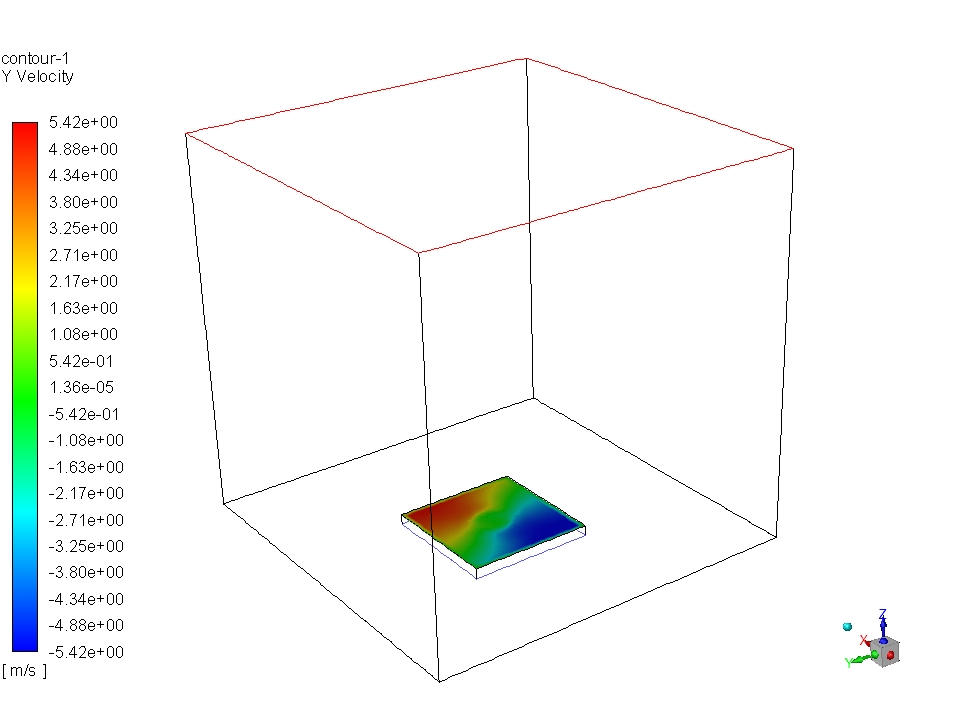Below are the contours for tangential velocity. I'm not sure if this is the right way to present tangential velocity contour. Perhaps, I'm wrong but I still expect to see values somewhere in the contour close to my input value.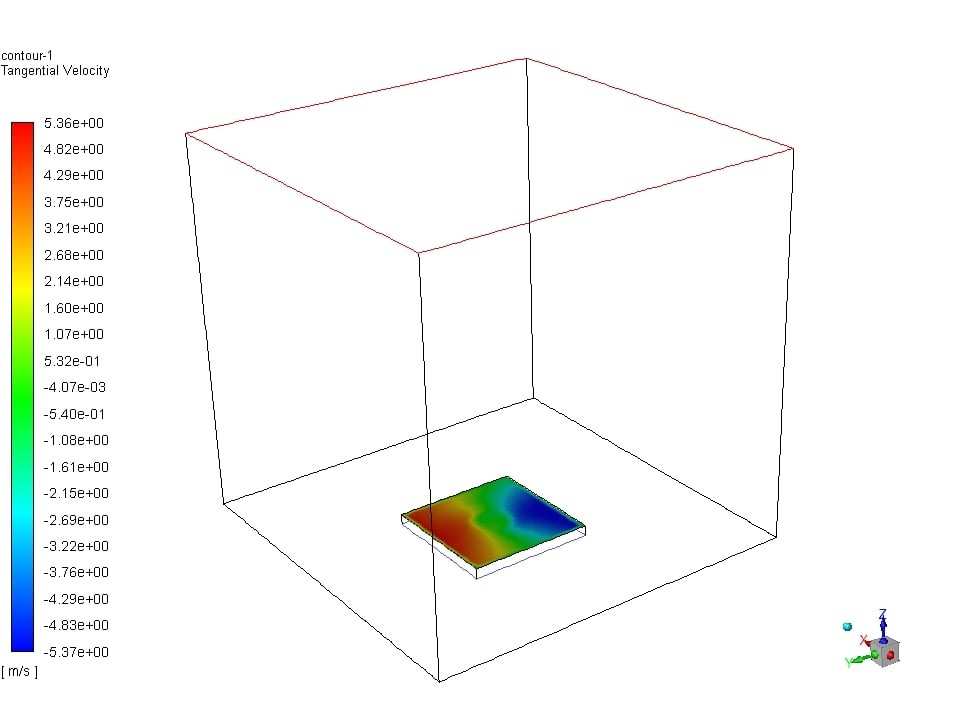•Rob
Ansys Employee

Your input tangential velocity is based on the fan axis & origin.  Your post processed velocity is based on the fluid coordinate which is has an origin of 0, 0, 0 and axis in +z by default.

Open the cell zone panel and click Help: you're looking for the section on specifying the rotation axis.

•erdenh
Subscriber

Thank you for helping me figure out how to plot the tangential velocity based on fan axis and origin.

Below is the updated tangential velocity contour.

However, I still cannot explain the difference between the maximum values in this contour plot and my input.

•erdenh
Subscriber

I used a milder constant tangential velocity (1 m/s instead of 13.74 m/s initially) this time. According to the plot of tangential velocity as a function of y direction along the midline of the fan surface, the values plateaued at around 0.5 m/s outside hub (half of the input). How should I interpret this?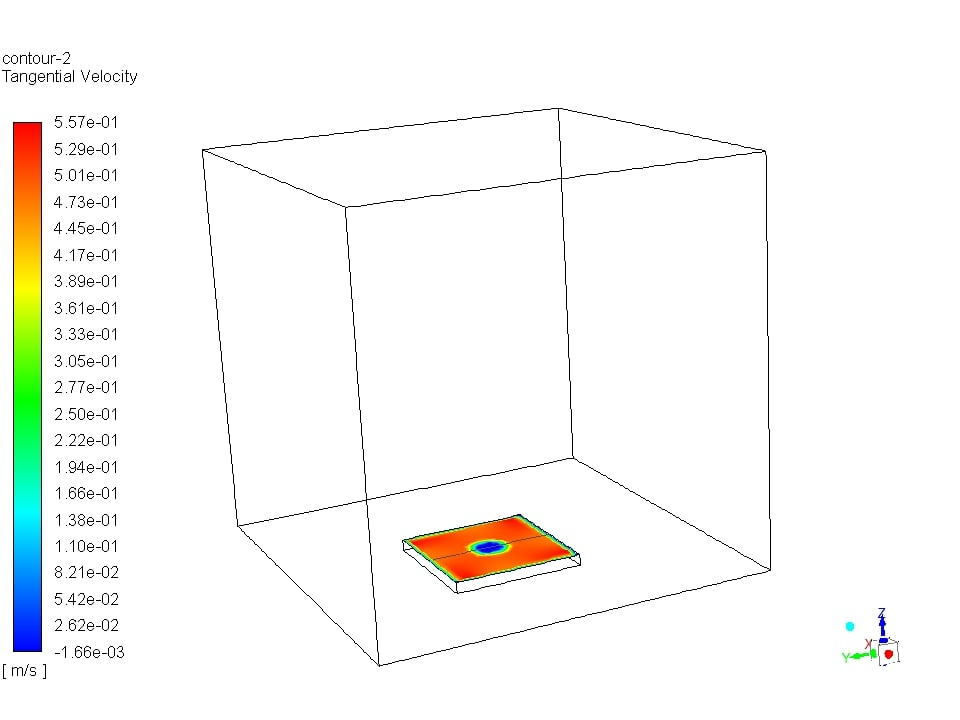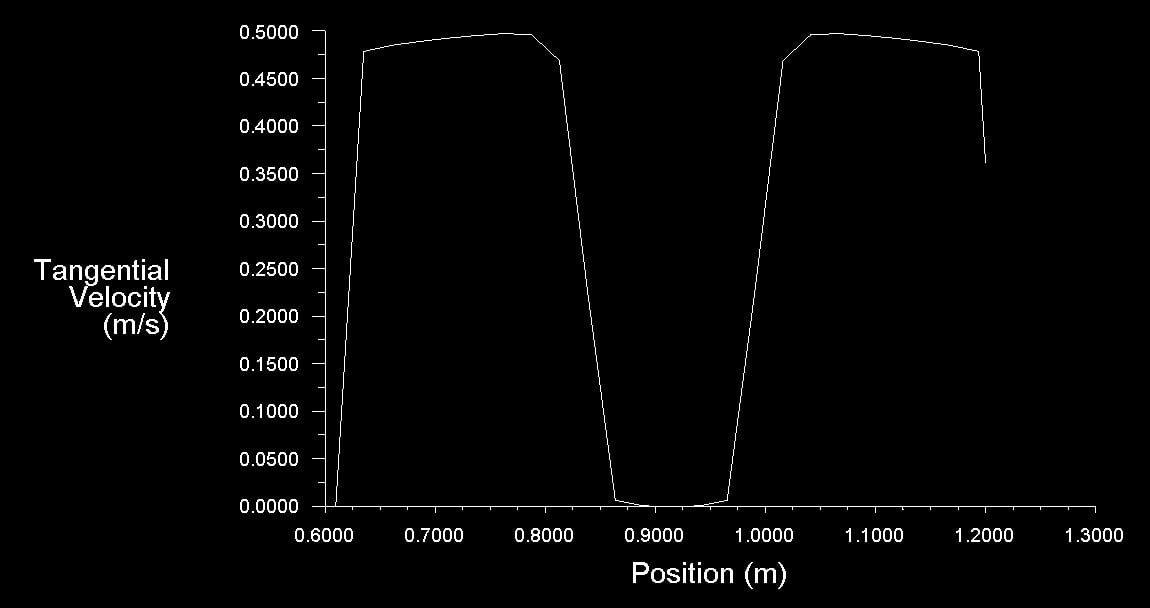•DrAmine
Ansys Employee

Can you check the values for the contour-plot by enabling node values and verify if they are not far away from the the tangential velocity set.

•erdenh
Subscriber

Node values were enabled by default for all results presented above.

•DrAmine
Ansys Employee

Fluent post processing does not report the specified tangential velocity on the fan bc panel. The post processor computes the tangential velocity from local cell conditions using the axis and origin defined in the cell zone panel. However, the tangential velocity specified in the boundary condition panel is used to introduce swirl in the flow by the fan model in the solver. The extent of the introduced swirl in the flow is determined by the difference of the specified swirl (tangential/radial velocity components) and the flow conditions encountered by the fan model. This in general will not give you exactly the imposed value but values in the vicinity of the specified swirl.

•erdenh
Subscriber

The values I see are in the vicinity of the half of the specified tangential velocity input. Is there a reason behind this?

•Rob
Ansys Employee

I think someone would need to have a look at the case to see what's going on. However, ANSYS staff are not permitted to review these files so we'd need to rely on the community to assist.

•erdenh
Subscriber

Any help from the community would be appreciated.

•uldoa
Subscriber

Hi, did you find a solution to your problem? I am having the same problem with tangential velocity beeing too low when using a pressure inlet.

•erdenh
Subscriber

Yes, the fan BC in Fluent seems to impose the tangential velocity within the first cell downstream of the fan. Since I was looking at the immediate surface where the fan bc is, I was reading values lower than the imposed tangential velocity.

•dhruvsuri
Subscriber

Hi, I'm trying to simulate a 2D actuator disk using reverse fan boundary condition similar to the image below. Could you help me with the implementation?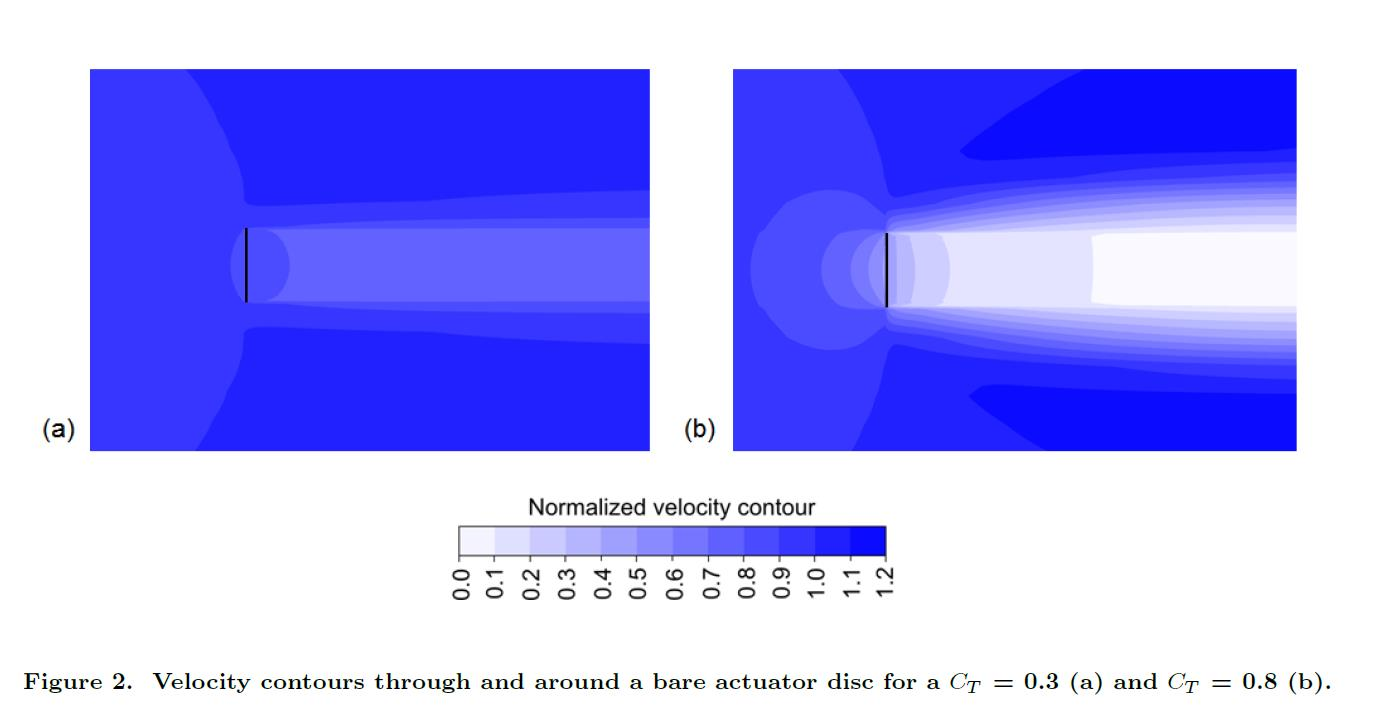I'd really appreciate the help. Thank you for your time!

•erdenh
Subscriber

If available, a negative pressure specification using fan boundary condition may help.